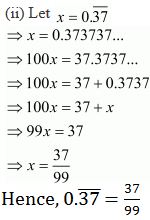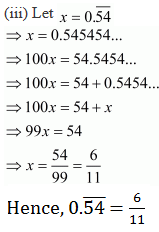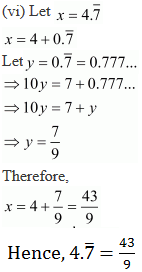#### Chapter 1 Number System R.D. Sharma Solutions for Class 9th Math Exercise 1.3

Exercise 1.3

1. Express each of the following decimals in the form p/q :
(i) 0.39
(ii) 0.750
(iii) 2.15
(iv) 7.010
(v) 9.90
(vi) 1.0001

Solution

(i) Given decimal is 0.39
Now we have to convert given decimal number into the p/q form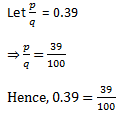(ii) Given decimal is 0.750
Now we have to convert given decimal number into p/q form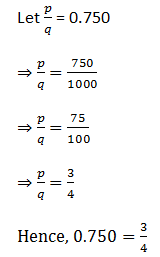(iii) Given decimal is 2.15
Now we have to express the given decimal number into p/q form(v) Given decimal is 9.90
Now we have to find given decimal number into p/q form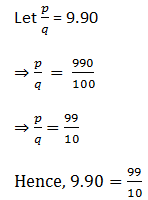(vi) Given decimal is 1.0001

Now we have to find given decimal number into p/q form2. Express each of the following decimals in the form p/q.Solution